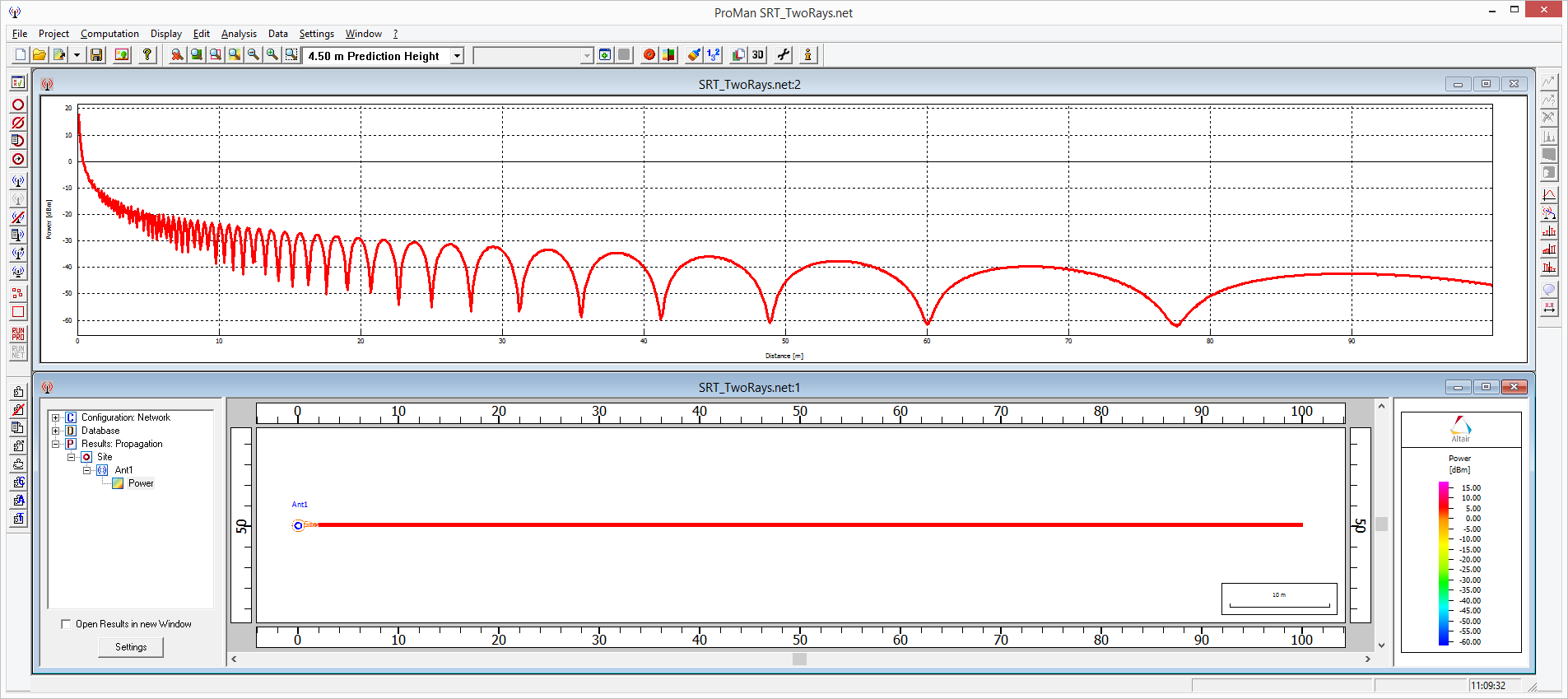## Recommended Posts

Hello everyone

As a part of some simulations I am running, I tried to simulate a 2-ray path model in ProMan. To achieve this I approximated a PEC in WallMan ( εr =1 (lowest possible),  µr = 1,  σ = 6e+006 (highest possible)) as a floor plate. In ProMan I simulated an antenna at one end (height 4.5m) and I plotted the received power vs the distance at height 4.5m. Everything about this graph is correct, the locations of the oscillations are exactly where I would suspect them theoretically and once  Δ R drops below  λ /2 the oscillations stop and I get a constant slope. However due to the small incidence angle and the relative height of the transmitter and respective receiver, I would predict the path loss of this slope to be 4 (40 dB/decade decrease, which I should get regardless of material properties because the angle is so small and I only have the direct ray and the reflected ray). But I never get this value from the simulations. The lower I place my transmitter, the lower the path loss gets. The path loss from every simulation has been lower then the expected value. Does anyone know the reason for this deviation or is someone struggling with the same phenomena?

I tried to look for an answer in the manual and found a possible cause for my mismatch. I suspect that my tolerance for the angle of incidence is to low. Therefore ProMan has trouble correctly interfering these two rays. I found a page in the manual, from which I have included a print screen, where oen could adjust this value. However I haven't been able to locate this menu for my simulations.

Kind regards

Publi##### Share on other sites

Hello,

the breakpoint describes the physical phenomenon that from a certain distance on the received power decreases with 40∙log(distance) instead of 20∙log(distance) which is valid for the free space propagation. This is due to the superposition of the direct ray with a ground reflected contribution.

The theoretical value of the breakpoint distance is calculated according to 4∙PI∙hTx∙hRx/λ.

So depending on the used frequency you can compute the breakpoint distance in your case, e.g. for 2 GHz with 4.5m height for Tx and Rx the breakpoint distance is 1696m, i.e. far beyond the shown minima in the below figure (first 100m). Basically the increased propagation exponent after the breakpoint is because the last minima is asymptotically approached.##### Share on other sites

When I utilize your formula for the breakpoint I get 848 m (I used 1 GHz). I also understand the reason for these oscillations and the way the last minima is calculated (although I use the same formulae without Pi, but for my question this should not matter as we are discussing the part of the graph long after this point). However the problem I encounter is that after this last breakpoint the received power should decrease with  40∙log(distance) as you have stated, and I agree. But when I simulate using the same parameters the power after the last breakpoint does not decrease with 40∙log(distance) but instead it decreases with 16∙log(distance), as you can see in the attachment. The lower I place my antennas, the worse this deviation gets. Is this due to the fact that for very low incidence angles, ProMan can no longer make the distinction between 2 rays that are actually direct path and reflected ray?## Join the conversation

You can post now and register later. If you have an account, sign in now to post with your account.×   Pasted as rich text.   Paste as plain text instead

Only 75 emoji are allowed.